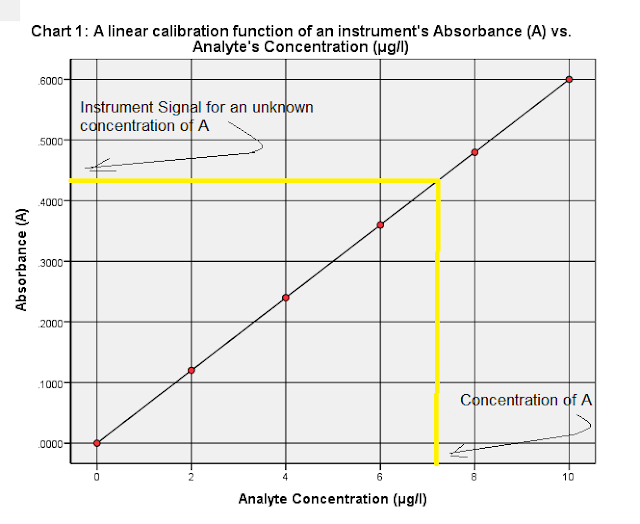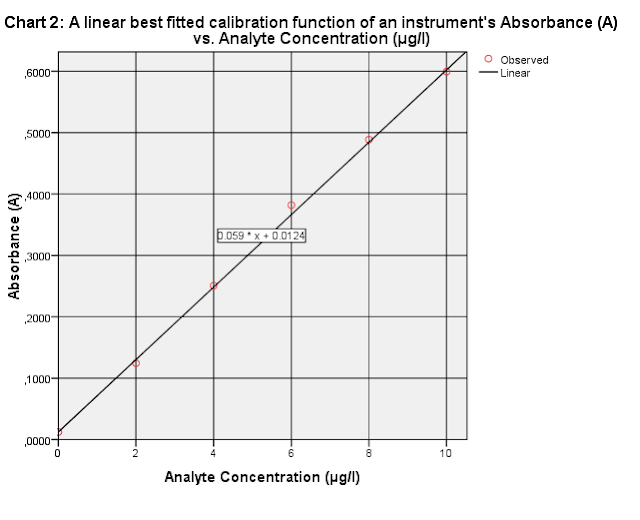What is calibration? Calibrated instruments|Analytical Chemistry | Chemistry Net

# What is calibration? Calibrated instruments|Analytical Chemistry

Why calibration is such a common and important step in analytical methods? How is defined?

Calibration is the process of assigning a value, usually in concentration units, to an instrument response.

For example, you might calibrate the response of an analytical device – such as a spectrophotometer - by analyzing different “known” concentrations of an analyte (i.e. solution of a metal ion such as Zn+2) and establish the instrument’s response per unit concentration of the metal (Fig. 1). Then, an unknown concentration of a test sample of the same analyte can be estimated from the graph by extrapolating the observed absorbance (y-axis) over to the analyte concentration (x-axis) (yellow line in Fig. 1).
In the graph shown in Fig. 1, the calibration function is established based on points in Table I.1:

Table I.1: Instrument Response for different analyte A  concentrations

 Analyte Concentration (μg/l) Instrument Response (Absorbance) 0 0 2 0.120 4 0.240 6 0.360 8 0.480 10 0.600

The line in Fig. 1 is called calibration line or in general calibration curve.Fig.1: A calibration line of an instrument's absorbance A vs. Analyte's Concentration

Calibration curve is the graphical relationship between the known values, such as concentrations, of a series of calibration standards and their instrument response.

What is the definition of calibration?

A definition of calibration according to IUPAC1 is as follows:

Calibration in Analytical Chemistry is the operation that determines the functional relationship between measured values (signal intensities S at certain signal positions zi) and analytical quantities characterizing types of analytes qi and their amount (content, concentration) n. Calibration includes the selection of the model (its functional form), the estimation of the model parameters as well as the errors, and their validation.

How an instrument – analytical device is calibrated?

As mentioned above, normally a calibration graph of a standard A response versus different concentrations of the standard A is constructed.
The analyte –standard A - response  may be: i) absorbance in U.V spectrometers  and atomic absortption spectrophotometers  ii) peak area (or height) or peak area (height) ratios to an internal standard in chromatographic assays.
The relationship may be linear (a straight line) or curved.
In the past – before ready access to personal computers and graph constructing softwares – calibration curves were drawn on graph paper and the concentrations interpolated manually. At that time having a straight line made the task considerably easier and scientists tried to work within a “linear” region of the curve even for techniques that are not linear (fluorescence, ECD).
Even today the ideal case is a linear response that passes directly through the origin comparing to the non-linear calibrations since the equation of a line is exactly known and therefore errors are minimized .

In case that the relationship is linear will be of the form:

(Smeas)A = k * nA + Sreag      (1)

or

(Smeas)A = k * CA+ Sreag      (2)

Notice that the above equations are of the form: y = m * x + b  (equation of a straight line). That means that k is the slope m of the straight line and Sreag is the intercept b of the line – the point where the line crosses the y axis.
Where (Smeas)A is the measured signal of substance A, k is a proportionality constant, nA or CA are the moles or concentration respectively of analyte A and Sreag is the signal due to the reagents (constant error)2.

In the special case that Sreag = 0 then the straight line passes through zero as in Figure 1.
The equation of the calibration line –straight line – in Fig. 1 is given by:

(Smeas)A = k * CA+ Sreag   or

y = m * x + b             (3)

The slope of the line = m = k = Rise/Run = 0.6/10 = 0.06 (determined directly from the graph in Fig.1). The intercept b = 0

Therefore (3) becomes      y = 0.06 * x
If you plug in values of x (analyte’s concentration) from Table I.1 in equation (3) you will get the corresponding values of y (instrument response) shown in the Table.

Normally though, the points from which the calibration function is determined do not fit so well as presented above.
Let us consider that the instrument’s response to analyte’s A concentrations are those given in Table I.2. Notice that for the standard solution with no analyte present the instrument response was 0.0123 – this standard is called a blank.

A blank is a specimen that is intended to contain none of the analytes of interest and which is subjected to the usual analytical or measurement process to establish a zero baseline or background value.

Table I.2: Instrument Response for different analyte A  concentrations

 Analyte Concentration (μg/l) Instrument Response (Absorbance) 0 .0123 2 .1244 4 .2507 6 .3820 8 .4886 10 .5995

In a case like this it is always good practice to plot data before carrying out any statistical analysis (regression). Plot just a scatter plot (Figure 2 – a scatter plot shows only the points and not the best fitted line) using Excel or any statistical software (SPSS, Sigmaplot…).

Are there any outliers in the calibration function?

You will note from the graph in Fig. 2 that there is some scattering around the best-fitted line and the line crosses the y axis not at zero as before. This is the normal situation for experimental data.
Since the best-fitted line is affected considerably by outliers – introduce significant errors - we must check the graph for the presence of possible outliers.Fig. 2: Absorbance of analyte A vs. Analyte A Concentration (linear best fitted line)

An outlier is a result which is significantly different from the rest of the data set. In the case of calibration, an outlier would appear as a point which is well removed from the other calibration points.

There is no outlier in Fig 2, just some scattering that is normal for experimental data.

The effects outliers can have on the best fitted calibration line are leverage and bias.

For example an outlier at the extremes of the calibration range can change the position of the calibration line by tilting it upwards or downwards (Table I.2a, Fig 2a, ). The instrument response at analyte concentration 10 μg/l is such an outlier.

Table I.2a: Instrument Response for different analyte A  concentrations

 Analyte Concentration (μg/l) Instrument Response (Absorbance) 0 .0123 2 .1244 4 .2507 6 .3820 8 .4886 10 .9995

This outlier is said to have a high degree of leverage. Leverage affects both the slope and the intercept of the calibration line and introduces errors.

An outlier in the middle of the calibration range will shift the best fitted line up or down. The outlier in this case introduces a bias into the position of the line – an additive error. The slope of the line will not be affected though but the intercept will be wrong.

Fig. 2a: Absorbance of analyte A vs. Analyte A Concentration (linear best fitted line). The outlier point (white point at (10, 0.9995)) that corresponds to analyte concentration = 10 μg/l was excluded when the best-fitted line was drawn (black line). When this outlier point is included in the calculations for the best fitted line the line changes position and the slope and intercept change considerably (red line).

How do you correct for outliers in the calibration graph?

Use one of the following methods, preferably the last three:

1)   Remove with caution points that are significantly different from the rest of the data. For example the outlier point in Fig. 2a can be excluded and the best fitted line should be drawn through the rest of the points.
2)    Repeat the calibration procedure and get the average of 5 or more replicate measurements at each concentration level (concentration levels ≥ 3). Then plot the averages of these replicate measurements at each concentration level.
3)      Use the method of standard additions.
4)      Use the bracketing method.
Then draw the best fitted calibration line checking first if the calibration is linear.

References

1.  K. Danzer, L.A. Currie, Pure & Appl. Chem., Vol. 70, 4, 993-1014 (1998)
2.  D. Harvey,  “Modern Analytical Chemistry”, McGraw-Hill Companies Inc., 2000
4.    A. Field, “Discovering Statistics using SPSS” , Sage Publications Ltd., 2005

1.2.